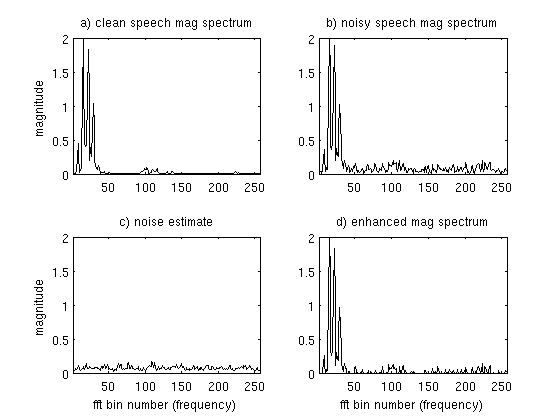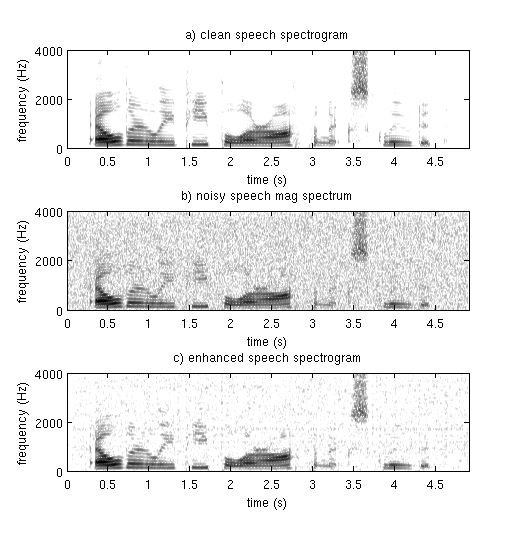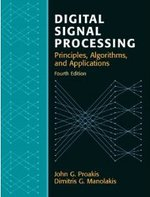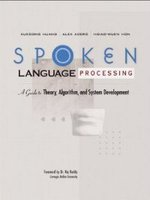# Speech Enhancement tutorial: Spectral Subraction

First we'll define our objective in speech enhancement: Given a noisy speech file, we wish to remove background noise to make the speech sound nicer, while not removing any of the speech. 'Nicer' is obviously a highly subjective term, usually what we go for is 'noise suppression'. Speech enhancement as a whole is a very large field, so on this page we'll go through one of the simplest algorithms: Spectral subtraction. For a more complete description of the field, try Speech Enhancement: Theory and Practice by Loizou, it is one of the best books on the topic.

Spectral subtraction was one of the first algorithms proposed for speech enhancement, with more papers being written about it than any other algorithm. The basic principle is as follows: if we assume additive noise, then we can subtract the noise spectrum from the noisy speech spectrum, so we are left with what should look like the clean speech spectrum. For this we need to know what the noise spectrum looks like, so we estimate it during regions of no speech (parts of the signal that contain only noise) and then assume it won't change much from frame to frame.

## A Detailed Explanation §

Assume we have a clean time domain signal, to which we add additive noise, . The signal we actually see is the sum . We wish to estimate the true value of given only .

The first step in spectral subtraction is to frame the speech signal into short, overlapping frames. Typically frames are taken to be about 20ms long. For a 16kHz sampled audio file, this corresponds to 0.020s * 16,000 samples/s = 400 samples in length. We then use an overlap of 50%, or about 200 samples. This means the first frame starts at sample 0, the second starts at sample 200, the third at 400 etc.

We would usually window each frame using e.g. the hamming window. We then take the discrete fourier transform of each frame and extract the magnitude and phase spectrum from each. A short aside on notation: we call our time domain signal . Once it is framed we have where n ranges over 1-400 (if our frames are 400 samples) and ranges over the number of frames. When we calculate the complex DFT, we get - where the denotes the frame number corresponding to the time-domain frame. is then the magnitude spectrum of frame .

To take the Discrete Fourier Transform of the frame, perform the following:

where is an sample long analysis window (e.g. hamming window), and is the length of the DFT. The magnitude spectrum for the speech frame is given by:

The phase spectrum is calculated in the following way:

In matlab, this would be done like so:

```windowed_frame = frame .* hamming(length(frame));
complex_spec = fft(windowed_frame,512);        % use a 512 point fft
mag_spec = abs(complex_spec);
phase_spec = angle(complex_spec);
```

We will be modifying mag_spec, but we will leave phase_spec alone, we will need to save it for later. If we apply the above steps to every frame, we should have lots of magnitude spectra. A common assumption is that the first few frames of an audio signal consist of silence, so they should be good examples of the noise spectrum. To get our noise estimate, we can take the mean of the first 3 or so frames. The more frames you use, the better your noise estimate will be, but you have to be careful not to get any speech frames included.

Now that we have the magnitude for each frame and a noise estimate, we can proceed with the meat of spectral subtraction: subtracting the noise estimate. This is done in the following way (here we use to represent the estimated clean spectrum, for the noisy spectrum we actually see, and for our noise estimate):

Note that we restrict our estimated magnitude spectrum to be positive, since magnitude spectrums must be. In matlab this is done as follows:

```clean_spec = mag_spec - noise_est;
clean_spec(clean_spec < 0) = 0;
```

Now we have an estimate of the clean spectrum for every frame. With this, we would like to reconstruct the original audio recording which will hopefully have less background noise. For this we use the clean spectrum and the phase spectrum from each frame that we calculated at the beginning, .

where and is our estimate of the clean complex spectrum for frame . In Matlab this would look like:

```enh_spec = clean_spec.*exp(j*phase_spec)
```

Now we need to do the IFFT (inverse FFT) of and do overlap add of the resulting time-domain frames to reconstruct our original signal. That is pretty much all there is to spectral subtraction, though there are many slight modifications that change the quality of the resulting enhanced speech.

## Modifications §

In the discussion above, we have defined magnitude spectral subtraction. Another closely related concept is power spectral subtraction, which we can see if we write the subtraction equation like this:

For we have magnitude spectral subtraction, but for we have power spectral subtraction. Alternatively you could have or . Each of these will have slightly different characteristics as far as noise suppression vs. loss of speech information goes.

Another modification is to use oversubtraction:

So far we have used , but if we felt like not enough noise was being removed we could use e.g. . This would result in more noise being removed, but this will probably also remove some of the speech information.

## Some Pictures to clarify things §

Here we have a set of plots showing the original clean spectrum (which normally we would never observe), noisy magnitude spectrum , our noise estimate , the estimated clean spectrum .

Here we have the spectrograms showing the original clean signal, the noisy signal and the enhanced signal. Notice that the enhanced signal does not look exactly like the original clean frame, this is because our noise estimate does not perfectly match the noise in the frame. This will always be a problem since noise is, by definition, unpredictable. The following plots show only the first half of the FFT.An example of Spectral Subtraction operating on a single frame

Below we have the spectrograms for clean speech, noisy speech and the enhanced speech. Note that the speech in the enhanced signal does not look the same as the speech in the clean spectrogram. This is because some of the low energy speech information has been lost. In general a speech enhancement algorithm will try and balance loss of speech vs. amount of background noise remaining.Spectrograms for clean, noisy and enhanced speech.

Hopefully this tutorial helps you understand spectral subtraction a little better, if you have any questions leave a comment below.

comments powered by Disqus

## Further reading

We recommend these books if you're interested in finding out more.Digital Signal Processing (4th Edition) ASIN/ISBN: 978-0131873742 A comprehensive guide to DSP Buy from Amazon.comSpoken Language Processing: A Guide to Theory, Algorithm and System Development ASIN/ISBN: 978-0130226167 A good overview of speech processing algorithms and techniques Buy from Amazon.com
Y NGP'I ZPGO AVCE GE LGM AVCE VJ OSCC VJ Y JAGMCN CYZS; VPN Y CYZS CSJJ IAVP AVCE GE LGM AVCE VJ OSCC VJ LGM NSJSUDS